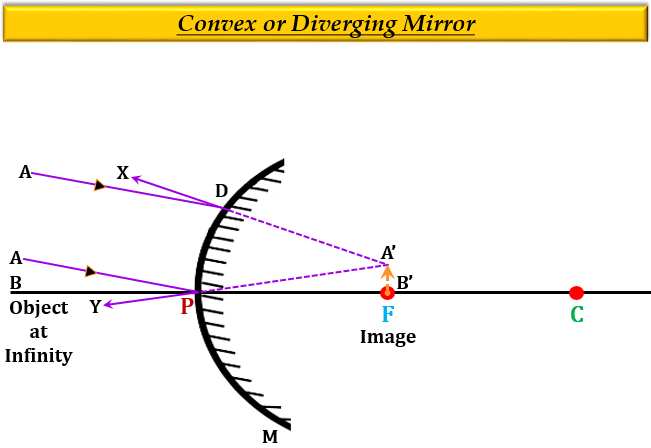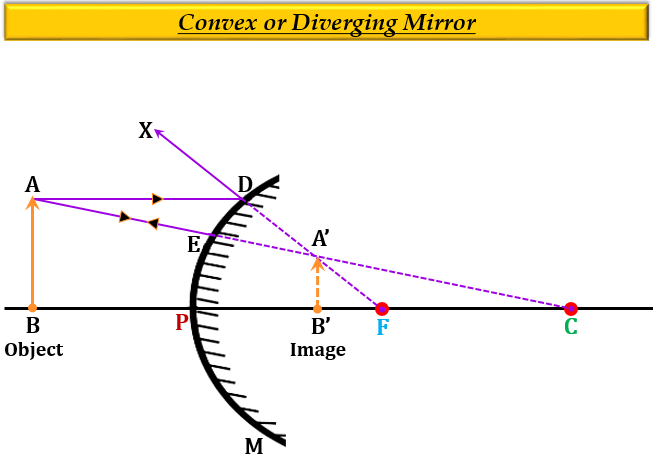# Draw ray diagrams showing the image formation by a convex mirror when an object is placed(a) at infinity(b) at finite distance from the mirror

$(a)$. Formation of image when the object is placed at infinity.$(b)$. Formation of image when the object is placed somewhere between infinity and the pole: